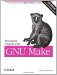# B.2 Arithmetic

 In the previous section, I noted that it is not possible to perform arithmetic in make using only its native features. I then showed how you could implement a simple counter by appending words to a list and returning the length of the list. Soon after I discovered the increment trick, Michael Mounteney posted a cool trick for performing a limited form of addition on integers in make . His trick manipulates the number line to compute the sum of two integers of size one or greater. To see how this works, imagine the number line: ` 2 3 4 5 6 7 8 9 10 11 12 13 14 15 ` Now, notice that (if we could get the subscripts right), we could add, say 4 plus 5, by first taking a subset of the line from the fourth element to the end then selecting the fifth element of the subset. We can do this with native make functions: ` number_line = 2 3 4 5 6 7 8 9 10 11 12 13 14 15 plus = \$(word , \$(wordlist , 15, \$(number_line))) four+five = \$(call plus, 4, 5) ` Very clever, Michael! Notice that the number line starts with 2 rather than 0 or 1. You can see that this is necessary if you run the plus function with 1 and 1. Both subscripts will yield the first element and the answer must be 2, therefore, the first element of the list must be 2. The reason for this is that, for the word and wordlist functions, the first element of the list has subscript 1 rather than (but I haven't bothered to prove it). Now, given a number line, we can perform addition, but how do we create a number line in make without typing it in by hand or using a shell program? We can create all numbers between 00 and 99 by combining all possible values in the tens place with all possible values in the ones place. For example: ` make -f - <<< '\$(warning \$(foreach i, 0 1 2, \$(addprefix \$i, 0 1 2)))' /c/TEMP/Gm002568:1: 00 01 02 10 11 12 20 21 22 ` By including all digits 0 through 9, we would produce all numbers from 00 to 99. By combining the foreach again with a hundreds column, we would get the numbers from 000 to 999, etc. All that is left is to strip the leading zeros where necessary. Here is a modified form of Mr. Mounteney's code to generate a number line and define the plus and gt operations: ` # combine - concatentate one sequence of numbers with another combine = \$(foreach i, , \$(addprefix \$i, )) # stripzero - Remove one leading zero from each word stripzero = \$(patsubst 0%,%,) # generate - Produce all permutations of three elements from the word list generate = \$(call stripzero, \ \$(call stripzero, \ \$(call combine, , \ \$(call combine, , )))) # number_line - Create a number line from 0 to 999 number_line := \$(call generate,0 1 2 3 4 5 6 7 8 9) length := \$(word \$(words \$(number_line)), \$(number_line)) # plus - Use the number line to add two integers plus = \$(word , \ \$(wordlist , \$(length), \ \$(wordlist 3, \$(length), \$(number_line)))) # gt - Use the number line to determine if is greater than gt = \$(filter , \ \$(wordlist 3, \$(length), \ \$(wordlist , \$(length), \$(number_line)))) all: @echo \$(call plus,4,7) @echo \$(if \$(call gt,4,7),is,is not) @echo \$(if \$(call gt,7,4),is,is not) @echo \$(if \$(call gt,7,7),is,is not) ` When run, the makefile yields: ` \$ make 11 is not is is not ` We can extend this code to include subtraction by noting that subscripting a reversed list is just like counting backwards . For example, to compute 7 minus 4, first create the number line subset 0 to 6, reverse it, then select the fourth element: ` number_line := 0 1 2 3 4 5 6 7 8 9... 1through6 := 0 1 2 3 4 5 6 reverse_it := 6 5 4 3 2 1 0 fourth_item := 3 ` Here is the algorithm in make syntax: ` # backwards - a reverse number line backwards := \$(call generate, 9 8 7 6 5 4 3 2 1 0) # reverse - reverse a list of words reverse = \$(strip \ \$(foreach f, \ \$(wordlist 1, \$(length), \$(backwards)), \ \$(word \$f, ))) # minus - compute minus minus = \$(word , \ \$(call reverse, \ \$(wordlist 1, , \$(number_line)))) minus: # \$(call minus, 7, 4) ` Multiplication and division are left as an exercise for the reader.Managing Projects with GNU Make (Nutshell Handbooks)
ISBN: 0596006101
EAN: 2147483647
Year: 2003
Pages: 131